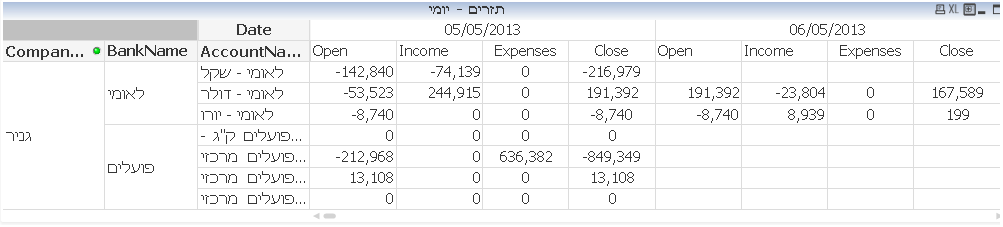# QlikView App Dev

Discussion Board for collaboration related to QlikView App Development.

Announcements
Join this live chat April 6, 10AM EST - QlikView to Qlik Sense REGISTER
cancel
Showing results for
Did you mean:Not applicable

## Formula outcome missing if no value to calculate

Hallo,

I'm having a problem with a balance sheet.

The balance is designed in this Structure: Open, Expenses, Income, Close

Each of this 4 fields is calculated for every day and the formulas are related to each other in the following way:Open = If(Date>Date(Today()-1),sum({\$<Day={\$(=Day(Today()-1))},

Month={\$(=Month(Today()-1))},

Year={\$(=Year(Today()-1))}>}

TOTAL<CompanyDesc,BankName,AccountName> CloseAmtCurr)

+ rangesum(Before(Sum({<TranType={'TranName'}>} TranAmtCurr),1,Columnno()))

+ rangesum(Before(Sum({<TranType={'TranName2'}>} TranAmtCurr),1,Columnno())),

if(Date=Date(Today()-1),Sum(CloseAmtCurr)))

Expenses=if(Date>=Today()-1,Sum ({\$<TranType={'TranName'}>}TranAmtCurr))

Income=if(Date>=Today()-1,Sum ({\$<TranType={'TranName2'}>}TranAmtCurr))

Close = if(Date>=Today()-1,[Open]+[Income]-[Expenses])

My problem is that when i have a date that does'nt incloud Expenses and Income the formula also omit the value of the Open and Close.

I want the open and close to be presented allways.

The logic of the Open and close should be like this:

(Today) Open = (Today-1) Close

(Today) Close = (Today) (Open + Income - Expenses)

I would Thank for any suggestion.

Thanks,

Uri

0 Replies